Ex 12.1

Chapter 12 Class 10 Surface Areas and Volumes
Serial order wise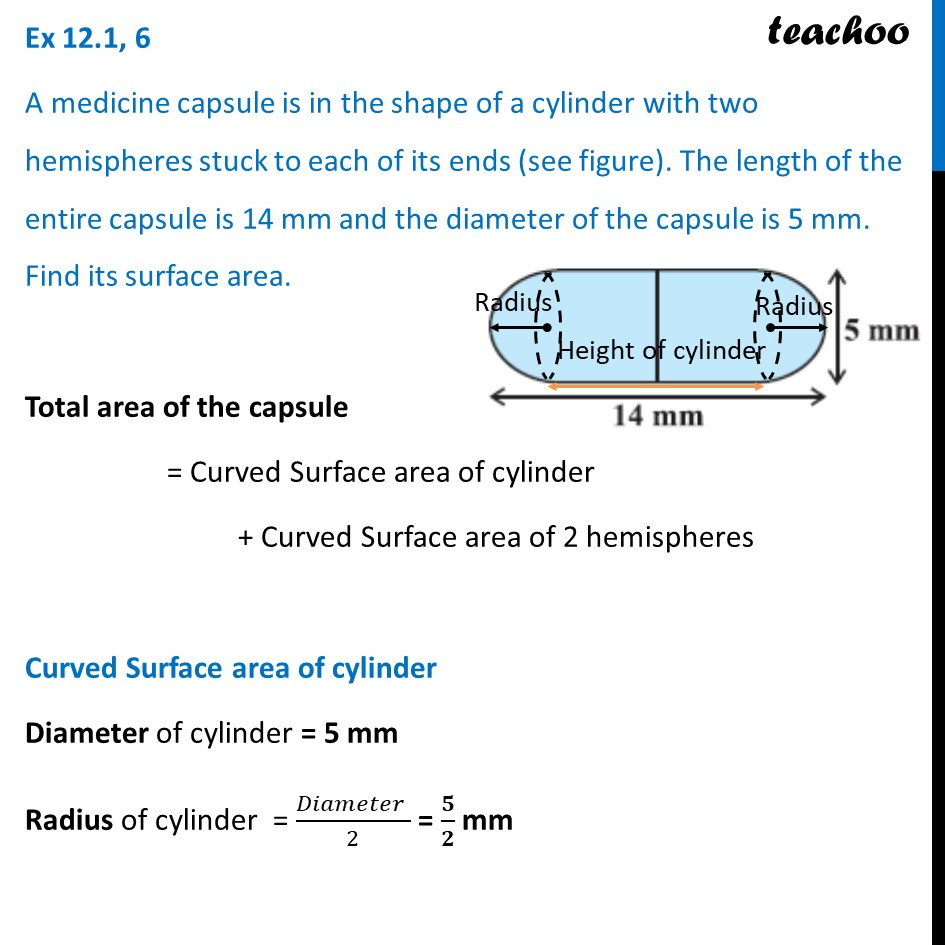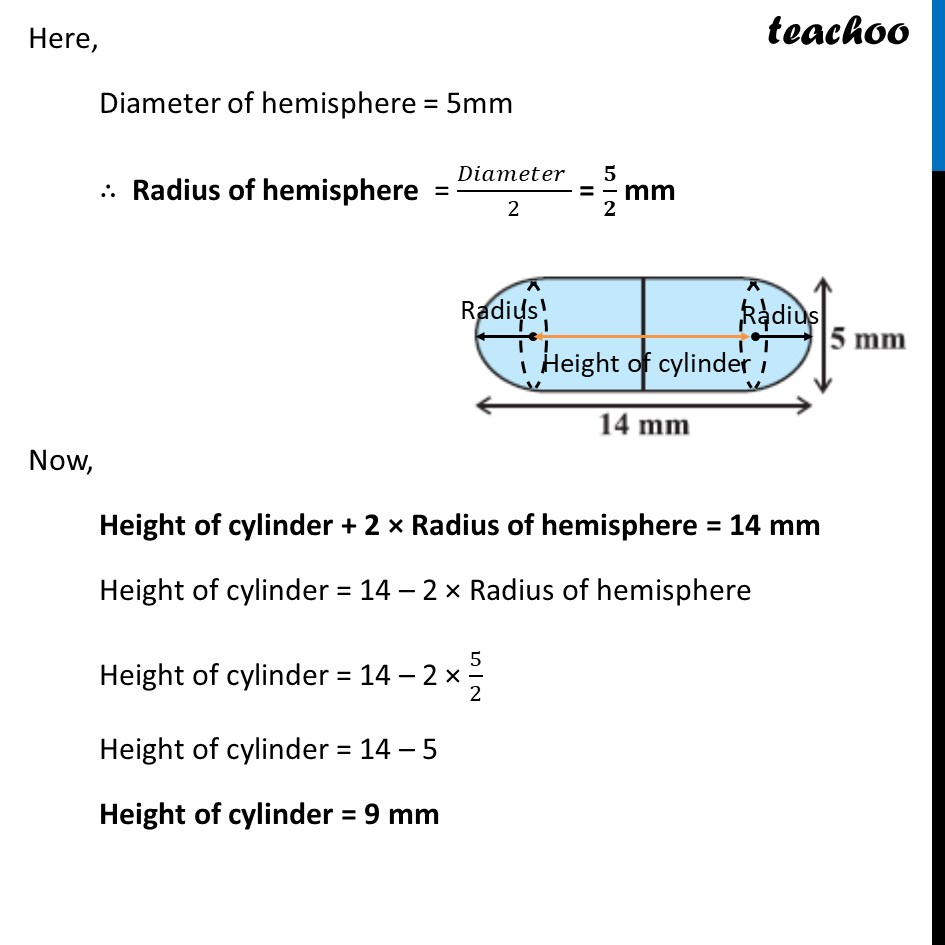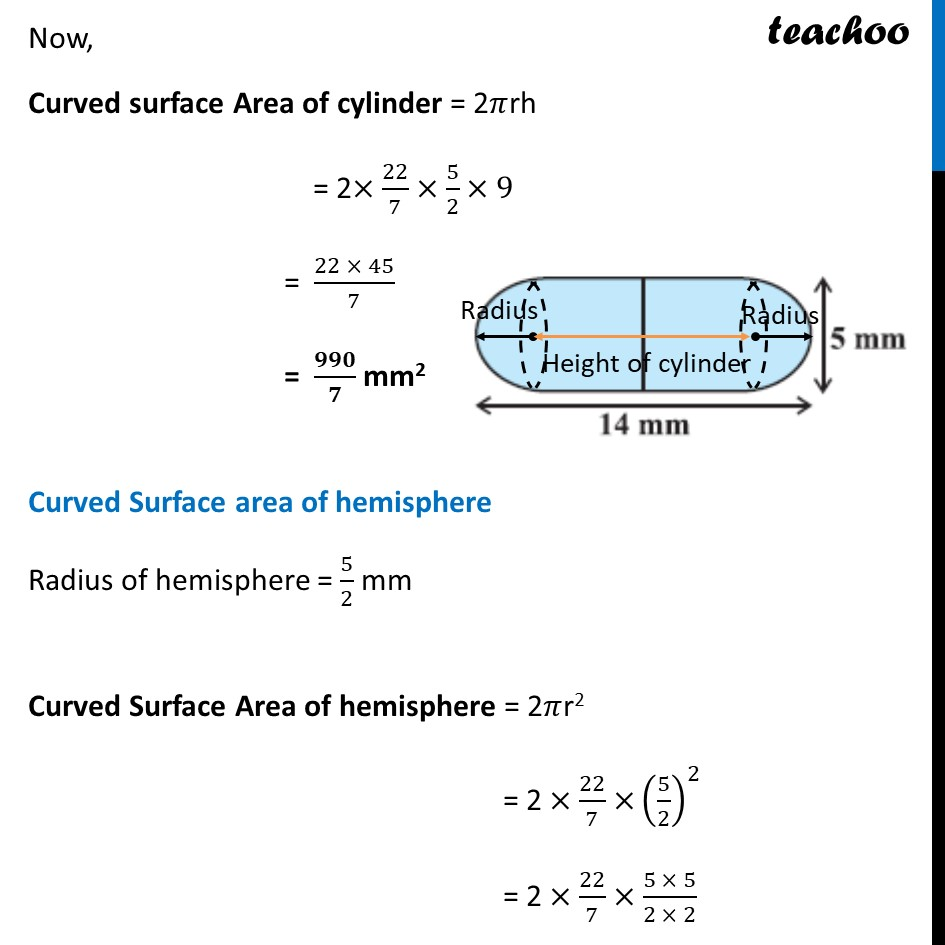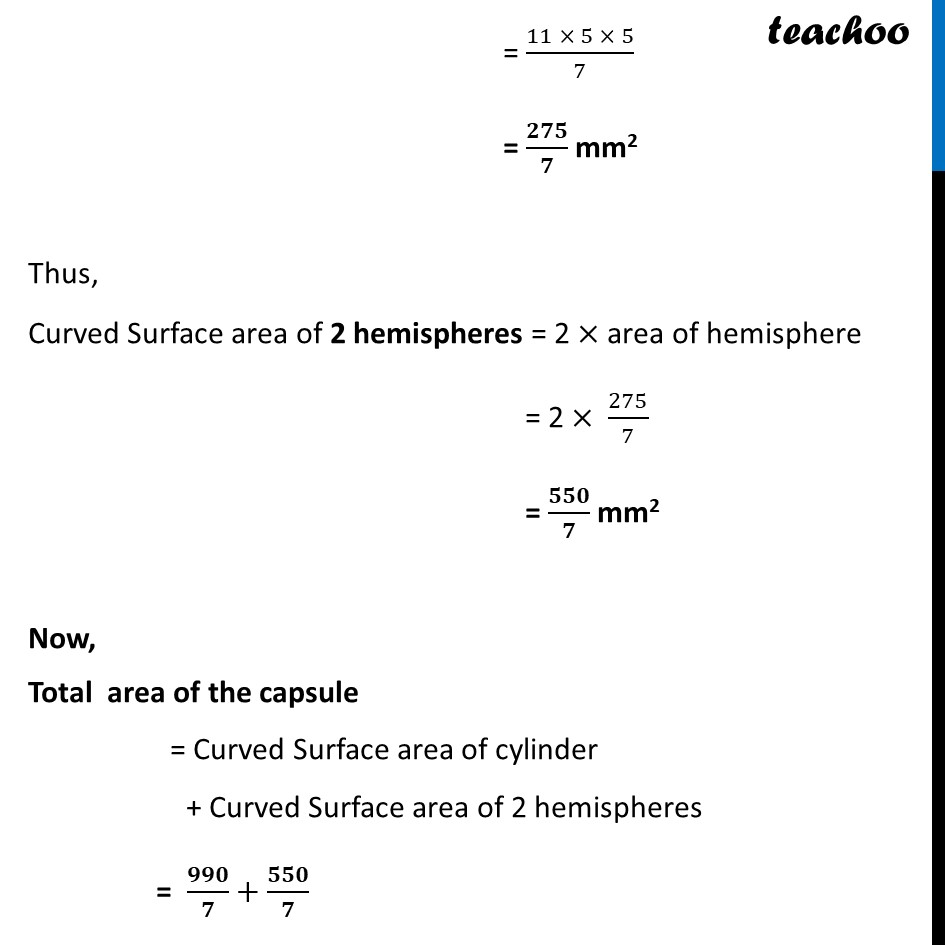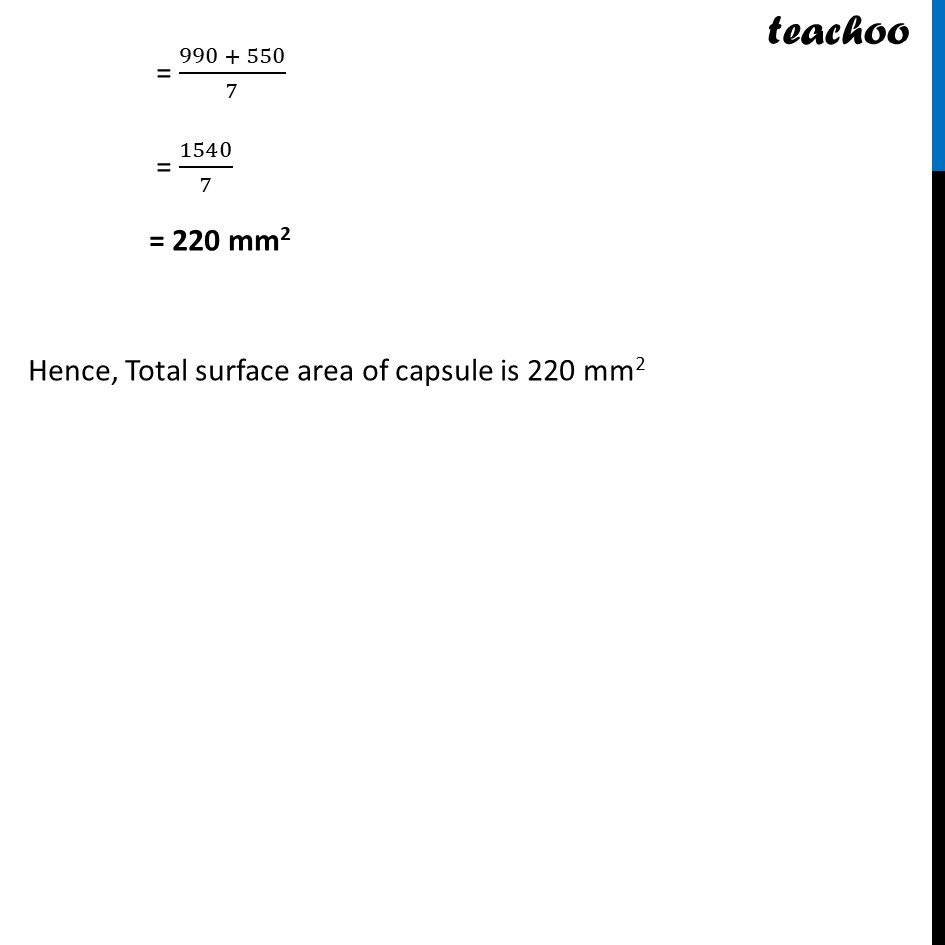Learn in your speed, with individual attention - Teachoo Maths 1-on-1 Class

### Transcript

Ex 12.1, 6 A medicine capsule is in the shape of a cylinder with two hemispheres stuck to each of its ends (see figure). The length of the entire capsule is 14 mm and the diameter of the capsule is 5 mm. Find its surface area. Total area of the capsule = Curved Surface area of cylinder + Curved Surface area of 2 hemispheres Curved Surface area of cylinder Diameter of cylinder = 5 mm Radius of cylinder = (𝐷𝑖𝑎𝑚𝑒𝑡𝑒𝑟 )/2 = 𝟓/𝟐 mm Here, Diameter of hemisphere = 5mm ∴ Radius of hemisphere = (𝐷𝑖𝑎𝑚𝑒𝑡𝑒𝑟 )/2 = 𝟓/𝟐 mm Now, Height of cylinder + 2 × Radius of hemisphere = 14 mm Height of cylinder = 14 – 2 × Radius of hemisphere Height of cylinder = 14 – 2 × 5/2 Height of cylinder = 14 – 5 Height of cylinder = 9 mm Now, Curved surface Area of cylinder = 2𝜋rh = 2×22/7×5/2×9 = (22 × 45)/7 = 𝟗𝟗𝟎/𝟕 mm2 Curved Surface area of hemisphere Radius of hemisphere = 5/2 mm Curved Surface Area of hemisphere = 2𝜋r2 = 2 ×22/7× (5/2)^2 = 2 ×22/7×(5 × 5)/(2 × 2) = (11 × 5 × 5)/7 = 𝟐𝟕𝟓/𝟕 mm2 Thus, Curved Surface area of 2 hemispheres = 2 × area of hemisphere = 2 × 275/7 = 𝟓𝟓𝟎/𝟕 mm2 Now, Total area of the capsule = Curved Surface area of cylinder + Curved Surface area of 2 hemispheres = 𝟗𝟗𝟎/𝟕+𝟓𝟓𝟎/𝟕 = (990 + 550)/7 = 1540/7 = 220 mm2 Hence, Total surface area of capsule is 220 mm2